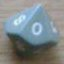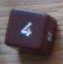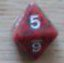Results in fraction instead of percentage

## Results in fraction instead of percentage

Return to topic list

 Thu Mar 27 23:50:34 2014   by   Daniel Its possible to troll tell me the result of a roll in fraction instead of percentage??Liked41 - 1/42 - 1/4etc... Fri Mar 28 15:34:31 2014   by   Torben I did try to make a version of Troll that used fractions, but I found that, quite often, the fractions would overflow if I used normal integers as numerators and denominators, even if I tried shortening the fractions.I might modify Troll to use fractions when possible and switch to reals when possible.  But it will probably not be very soon.I could, of course, show the reals as fractions, but, due to loss of precision, it would not always be possible to see what the exact fraction should be.  For example, if the exact fraction is 1/3, the inexact real number might be converted to 34296/1028929 (which is close to 1/3). Mon Oct 26 12:04:04 2015   by   Daniel Hi Torben,I need fractions again, so searching here I found my own topic, haha...It still difficult to modify Troll source code to add a fraction notation?It is possible to improvise it using a function? Mon Oct 26 13:27:06 2015   by   Torben Still no immediate plans of using fractions.  What you can do, however, is to set the multiplier to something other than 100.  For example, if you roll 3d6, you can set the multiplier to 216 (6×6×6) and get results as multiplas of 1/216, from 1/216 for 3 or 18 up to 27/216 for 10 or 11. Tue Oct 27 21:34:55 2015   by   Daniel Wow! I consider it "a definitive solution", don't you?If you implement a "fractions checkbox" that set the "multiplier" to [number of possible rolls] and "digits of precision" to zero, I think its is done, right?Anyway, thanks to solve my problem. Wed Oct 28 10:16:45 2015   by   Torben The number of possible rolls might be infinite, and even when it is not, the number of possible rolls might not be the correct denominator.For example, `d d10` will roll a d10 and then a dice with the number of sides shown on the d10. The number of possible rolls is 1+2+...+9+10 = 55, but 55 as a denominator does not give integral numerators.  In fact, you need 25200 as denominator to get integral fractions.Whenever a die is rolled depending on a result of a previous die, you can't use the number of possible rolls as denominator. Wed Nov 21 17:09:01 2018   by   Keith I could be barking up the wrong tree (3+ years later), but the LCM of the first 10 naturals is only 2520 (5x7x8x9 or 23 x 32 x 5 x 7). Wed Nov 21 17:52:05 2018   by   Keith Sorry my bad. Turns out the odds of getting a 1 is 7381/252000. Forgot about the first dice. However the odds of getting n is (10n)-1 + (10(n+1))-1, (10(n+2))-1 ...until you get to (10(10))-1 or 0.01. For instance the odds of getting a 7 is 1/70 + 1/80 + 1/90 + 1/100 = 0.04789682539682539682539682539683 or 1207/25200. Wed Nov 21 17:57:56 2018   by   Keith Sorry one to many zeros. It should be 25200.

Return to topic list

New message:
 Topic: Posted by:

Type the values of the dice shown below:Return to topic list